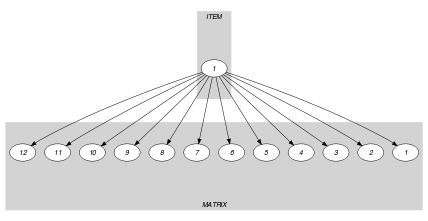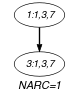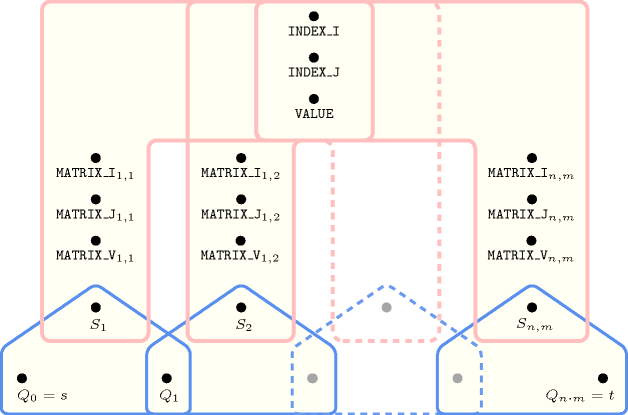## 5.142. element_matrix

Origin

CHIP

Constraint

$\mathrm{𝚎𝚕𝚎𝚖𝚎𝚗𝚝}_\mathrm{𝚖𝚊𝚝𝚛𝚒𝚡}\left(\mathrm{𝙼𝙰𝚇}_𝙸,\mathrm{𝙼𝙰𝚇}_𝙹,\mathrm{𝙸𝙽𝙳𝙴𝚇}_𝙸,\mathrm{𝙸𝙽𝙳𝙴𝚇}_𝙹,\mathrm{𝙼𝙰𝚃𝚁𝙸𝚇},\mathrm{𝚅𝙰𝙻𝚄𝙴}\right)$

Synonyms

$\mathrm{𝚎𝚕𝚎𝚖}_\mathrm{𝚖𝚊𝚝𝚛𝚒𝚡}$, $\mathrm{𝚖𝚊𝚝𝚛𝚒𝚡}$.

Arguments
 $\mathrm{𝙼𝙰𝚇}_𝙸$ $\mathrm{𝚒𝚗𝚝}$ $\mathrm{𝙼𝙰𝚇}_𝙹$ $\mathrm{𝚒𝚗𝚝}$ $\mathrm{𝙸𝙽𝙳𝙴𝚇}_𝙸$ $\mathrm{𝚍𝚟𝚊𝚛}$ $\mathrm{𝙸𝙽𝙳𝙴𝚇}_𝙹$ $\mathrm{𝚍𝚟𝚊𝚛}$ $\mathrm{𝙼𝙰𝚃𝚁𝙸𝚇}$ $\mathrm{𝚌𝚘𝚕𝚕𝚎𝚌𝚝𝚒𝚘𝚗}\left(𝚒-\mathrm{𝚒𝚗𝚝},𝚓-\mathrm{𝚒𝚗𝚝},𝚟-\mathrm{𝚒𝚗𝚝}\right)$ $\mathrm{𝚅𝙰𝙻𝚄𝙴}$ $\mathrm{𝚍𝚟𝚊𝚛}$
Restrictions
 $\mathrm{𝙼𝙰𝚇}_𝙸\ge 1$ $\mathrm{𝙼𝙰𝚇}_𝙹\ge 1$ $\mathrm{𝙸𝙽𝙳𝙴𝚇}_𝙸\ge 1$ $\mathrm{𝙸𝙽𝙳𝙴𝚇}_𝙸\le \mathrm{𝙼𝙰𝚇}_𝙸$ $\mathrm{𝙸𝙽𝙳𝙴𝚇}_𝙹\ge 1$ $\mathrm{𝙸𝙽𝙳𝙴𝚇}_𝙹\le \mathrm{𝙼𝙰𝚇}_𝙹$ $\mathrm{𝚛𝚎𝚚𝚞𝚒𝚛𝚎𝚍}$$\left(\mathrm{𝙼𝙰𝚃𝚁𝙸𝚇},\left[𝚒,𝚓,𝚟\right]\right)$ $\mathrm{𝚒𝚗𝚌𝚛𝚎𝚊𝚜𝚒𝚗𝚐}_\mathrm{𝚜𝚎𝚚}$$\left(\mathrm{𝙼𝙰𝚃𝚁𝙸𝚇},\left[𝚒,𝚓\right]\right)$ $\mathrm{𝙼𝙰𝚃𝚁𝙸𝚇}.𝚒\ge 1$ $\mathrm{𝙼𝙰𝚃𝚁𝙸𝚇}.𝚒\le \mathrm{𝙼𝙰𝚇}_𝙸$ $\mathrm{𝙼𝙰𝚃𝚁𝙸𝚇}.𝚓\ge 1$ $\mathrm{𝙼𝙰𝚃𝚁𝙸𝚇}.𝚓\le \mathrm{𝙼𝙰𝚇}_𝙹$ $|\mathrm{𝙼𝙰𝚃𝚁𝙸𝚇}|=\mathrm{𝙼𝙰𝚇}_𝙸*\mathrm{𝙼𝙰𝚇}_𝙹$
Purpose

The $\mathrm{𝙼𝙰𝚃𝚁𝙸𝚇}$ collection corresponds to the two-dimensional matrix $\mathrm{𝙼𝙰𝚃𝚁𝙸𝚇}\left[1..\mathrm{𝙼𝙰𝚇}_𝙸,1..\mathrm{𝙼𝙰𝚇}_𝙹\right]$. $\mathrm{𝚅𝙰𝙻𝚄𝙴}$ is equal to the entry $\mathrm{𝙼𝙰𝚃𝚁𝙸𝚇}\left[\mathrm{𝙸𝙽𝙳𝙴𝚇}_𝙸,\mathrm{𝙸𝙽𝙳𝙴𝚇}_𝙹\right]$ of the previous matrix.

Example
$\left(\begin{array}{c}4,3,1,3,〈\begin{array}{ccc}𝚒-1\hfill & 𝚓-1\hfill & 𝚟-4,\hfill \\ 𝚒-1\hfill & 𝚓-2\hfill & 𝚟-1,\hfill \\ 𝚒-1\hfill & 𝚓-3\hfill & 𝚟-7,\hfill \\ 𝚒-2\hfill & 𝚓-1\hfill & 𝚟-1,\hfill \\ 𝚒-2\hfill & 𝚓-2\hfill & 𝚟-0,\hfill \\ 𝚒-2\hfill & 𝚓-3\hfill & 𝚟-8,\hfill \\ 𝚒-3\hfill & 𝚓-1\hfill & 𝚟-3,\hfill \\ 𝚒-3\hfill & 𝚓-2\hfill & 𝚟-2,\hfill \\ 𝚒-3\hfill & 𝚓-3\hfill & 𝚟-1,\hfill \\ 𝚒-4\hfill & 𝚓-1\hfill & 𝚟-0,\hfill \\ 𝚒-4\hfill & 𝚓-2\hfill & 𝚟-0,\hfill \\ 𝚒-4\hfill & 𝚓-3\hfill & 𝚟-6\hfill \end{array}〉,7\hfill \end{array}\right)$

The $\mathrm{𝚎𝚕𝚎𝚖𝚎𝚗𝚝}_\mathrm{𝚖𝚊𝚝𝚛𝚒𝚡}$ constraint holds since its last argument $\mathrm{𝚅𝙰𝙻𝚄𝙴}=7$ is equal to the $𝚟$ attribute of the ${k}^{th}$ item of the $\mathrm{𝙼𝙰𝚃𝚁𝙸𝚇}$ collection such that $\mathrm{𝙼𝙰𝚃𝚁𝙸𝚇}\left[k\right].i=\mathrm{𝙸𝙽𝙳𝙴𝚇}_𝙸=1$ and $\mathrm{𝙼𝙰𝚃𝚁𝙸𝚇}\left[k\right].j=\mathrm{𝙸𝙽𝙳𝙴𝚇}_𝙹=3$.

Typical
 $\mathrm{𝙼𝙰𝚇}_𝙸>1$ $\mathrm{𝙼𝙰𝚇}_𝙹>1$ $|\mathrm{𝙼𝙰𝚃𝚁𝙸𝚇}|>3$ $\mathrm{𝚖𝚊𝚡𝚟𝚊𝚕}$$\left(\mathrm{𝙼𝙰𝚃𝚁𝙸𝚇}.𝚒\right)>1$ $\mathrm{𝚖𝚊𝚡𝚟𝚊𝚕}$$\left(\mathrm{𝙼𝙰𝚃𝚁𝙸𝚇}.𝚓\right)>1$ $\mathrm{𝚛𝚊𝚗𝚐𝚎}$$\left(\mathrm{𝙼𝙰𝚃𝚁𝙸𝚇}.𝚟\right)>1$
Symmetry

All occurrences of two distinct values in $\mathrm{𝙼𝙰𝚃𝚁𝙸𝚇}.𝚟$ or $\mathrm{𝚅𝙰𝙻𝚄𝙴}$ can be swapped; all occurrences of a value in $\mathrm{𝙼𝙰𝚃𝚁𝙸𝚇}.𝚟$ or $\mathrm{𝚅𝙰𝙻𝚄𝙴}$ can be renamed to any unused value.

Reformulation

The $\mathrm{𝚎𝚕𝚎𝚖𝚎𝚗𝚝}_\mathrm{𝚖𝚊𝚝𝚛𝚒𝚡}$$\left(\mathrm{𝙼𝙰𝚇}_𝙸,\mathrm{𝙼𝙰𝚇}_𝙹,\mathrm{𝙸𝙽𝙳𝙴𝚇}_𝙸,\mathrm{𝙸𝙽𝙳𝙴𝚇}_𝙹,\mathrm{𝙼𝙰𝚃𝚁𝙸𝚇},\mathrm{𝚅𝙰𝙻𝚄𝙴}\right)$ constraint can be expressed in term of $\mathrm{𝙼𝙰𝚇}_𝙸$ $\mathrm{𝚎𝚕𝚎𝚖𝚎𝚗𝚝}$$\left(\mathrm{𝙸𝙽𝙳𝙴𝚇}_𝙹,{\mathrm{𝙻𝙸𝙽𝙴}}_{i},{\mathrm{𝚅𝙰𝚁}}_{i}\right)$ $\left(i\in \left[1,\mathrm{𝙼𝙰𝚇}_𝙸\right]\right)$, where ${\mathrm{𝙻𝙸𝙽𝙴}}_{i}$ corresponds to the $i$-th line of the matrix $\mathrm{𝙼𝙰𝚃𝚁𝙸𝚇}$ and of one $\mathrm{𝚎𝚕𝚎𝚖𝚎𝚗𝚝}$$\left(\mathrm{𝙸𝙽𝙳𝙴𝚇}_𝙸,〈{\mathrm{𝚅𝙰𝚁}}_{1},{\mathrm{𝚅𝙰𝚁}}_{2},\cdots ,{\mathrm{𝚅𝙰𝚁}}_{\mathrm{𝙼𝙰𝚇}_𝙸}〉,\mathrm{𝚅𝙰𝙻𝚄𝙴}\right)$ constraint.

If we consider the Example slot we get the following $\mathrm{𝚎𝚕𝚎𝚖𝚎𝚗𝚝}$ constraints:

Systems

nth in Choco, element in Gecode.

Keywords
Derived Collection
$\mathrm{𝚌𝚘𝚕}\left(\begin{array}{c}\mathrm{𝙸𝚃𝙴𝙼}-\mathrm{𝚌𝚘𝚕𝚕𝚎𝚌𝚝𝚒𝚘𝚗}\left(\mathrm{𝚒𝚗𝚍𝚎𝚡}_𝚒-\mathrm{𝚍𝚟𝚊𝚛},\mathrm{𝚒𝚗𝚍𝚎𝚡}_𝚓-\mathrm{𝚍𝚟𝚊𝚛},\mathrm{𝚟𝚊𝚕𝚞𝚎}-\mathrm{𝚍𝚟𝚊𝚛}\right),\hfill \\ \mathrm{𝚒𝚝𝚎𝚖}\left(\mathrm{𝚒𝚗𝚍𝚎𝚡}_𝚒-\mathrm{𝙸𝙽𝙳𝙴𝚇}_𝙸,\mathrm{𝚒𝚗𝚍𝚎𝚡}_𝚓-\mathrm{𝙸𝙽𝙳𝙴𝚇}_𝙹,\mathrm{𝚟𝚊𝚕𝚞𝚎}-\mathrm{𝚅𝙰𝙻𝚄𝙴}\right)\right]\hfill \end{array}\right)$
Arc input(s)

$\mathrm{𝙸𝚃𝙴𝙼}$ $\mathrm{𝙼𝙰𝚃𝚁𝙸𝚇}$

Arc generator
$\mathrm{𝑃𝑅𝑂𝐷𝑈𝐶𝑇}$$↦\mathrm{𝚌𝚘𝚕𝚕𝚎𝚌𝚝𝚒𝚘𝚗}\left(\mathrm{𝚒𝚝𝚎𝚖},\mathrm{𝚖𝚊𝚝𝚛𝚒𝚡}\right)$

Arc arity
Arc constraint(s)
 $•\mathrm{𝚒𝚝𝚎𝚖}.\mathrm{𝚒𝚗𝚍𝚎𝚡}_𝚒=\mathrm{𝚖𝚊𝚝𝚛𝚒𝚡}.𝚒$ $•\mathrm{𝚒𝚝𝚎𝚖}.\mathrm{𝚒𝚗𝚍𝚎𝚡}_𝚓=\mathrm{𝚖𝚊𝚝𝚛𝚒𝚡}.𝚓$ $•\mathrm{𝚒𝚝𝚎𝚖}.\mathrm{𝚟𝚊𝚕𝚞𝚎}=\mathrm{𝚖𝚊𝚝𝚛𝚒𝚡}.𝚟$
Graph property(ies)
$\mathrm{𝐍𝐀𝐑𝐂}$$=1$

Graph model

Similar to the $\mathrm{𝚎𝚕𝚎𝚖𝚎𝚗𝚝}$ constraint except that the arc constraint is updated according to the fact that we have a two-dimensional matrix.

Parts (A) and (B) of Figure 5.142.1 respectively show the initial and final graph associated with the Example slot. Since we use the $\mathrm{𝐍𝐀𝐑𝐂}$ graph property, the unique arc of the final graph is stressed in bold.

##### Figure 5.142.1. Initial and final graph of the $\mathrm{𝚎𝚕𝚎𝚖𝚎𝚗𝚝}_\mathrm{𝚖𝚊𝚝𝚛𝚒𝚡}$ constraint(a)(b)
Signature

Because of the first condition of the arc constraint the final graph cannot have more than one arc. Therefore we can rewrite $\mathrm{𝐍𝐀𝐑𝐂}=1$ to $\mathrm{𝐍𝐀𝐑𝐂}\ge 1$ and simplify $\underline{\overline{\mathrm{𝐍𝐀𝐑𝐂}}}$ to $\overline{\mathrm{𝐍𝐀𝐑𝐂}}$.

Automaton

Figure 5.142.2 depicts the automaton associated with the $\mathrm{𝚎𝚕𝚎𝚖𝚎𝚗𝚝}_\mathrm{𝚖𝚊𝚝𝚛𝚒𝚡}$ constraint. Let ${𝙸}_{k}$, ${𝙹}_{k}$ and ${𝚅}_{k}$ respectively be the $𝚒$, the $𝚓$ and the $𝚟$ ${k}^{th}$ attributes of the $\mathrm{𝙼𝙰𝚃𝚁𝙸𝚇}$ collection. To each sextuple $\left(\mathrm{𝙸𝙽𝙳𝙴𝚇}_𝙸,\mathrm{𝙸𝙽𝙳𝙴𝚇}_𝙹,\mathrm{𝚅𝙰𝙻𝚄𝙴},{𝙸}_{k},{𝙹}_{k},{𝚅}_{k}\right)$ corresponds a 0-1 signature variable ${S}_{k}$ as well as the following signature constraint: $\left(\left(\mathrm{𝙸𝙽𝙳𝙴𝚇}_𝙸={𝙸}_{k}\right)\wedge \left(\mathrm{𝙸𝙽𝙳𝙴𝚇}_𝙹={𝙹}_{k}\right)\wedge \left(\mathrm{𝚅𝙰𝙻𝚄𝙴}={𝚅}_{k}\right)\right)⇔{S}_{k}$.

##### Figure 5.142.2. Automaton of the $\mathrm{𝚎𝚕𝚎𝚖𝚎𝚗𝚝}_\mathrm{𝚖𝚊𝚝𝚛𝚒𝚡}$ constraint##### Figure 5.142.3. Hypergraph of the reformulation corresponding to the automaton of the $\mathrm{𝚎𝚕𝚎𝚖𝚎𝚗𝚝}_\mathrm{𝚖𝚊𝚝𝚛𝚒𝚡}$ constraint where $n$ and $m$ respectively stands for $\mathrm{𝙼𝙰𝚇}_𝙸$ and $\mathrm{𝙼𝙰𝚇}_𝙹$